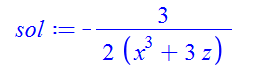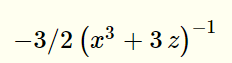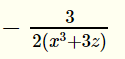:

## improving Latex output of Maple for expressions

Maple 2018

There are many questions that complain about Latex conversion in Maple.

I'd like to again request that Maplesoft improves Latex output of its expressions. If Maple can just fix how it generates fractions, that will good enough for now.

I am willing to send Maplesoft a personal check of the amount of one month salary for one of your developers to do this fix if you are willing to do it. It should not take more than one month to do this simple fix in your code. It might even take one day if someone knows the code.

The problem comes when there is a fraction in the expression. the Latex output instead of using proper latex code using "\frac{}{}", it instead uses "/" which makes the output terrible.

Another case, where Maple generate (expression)^{-1} instead of \frac{1}{expression}.

It can't be that hard to fix these 2 issues, which can go a long way towards making the latex generated by Maple much better. Here is an example

eq:=-(1/2)*1/y = (1/3)*x^3+z:
sol:=solve(eq,y);latex(sol);
-3/2\, \left( {x}^{3}+3\,z \right) ^{-1}

Which renders asWhich is terrible. The screen output is much better.

Compare this to Mathematica

eq = -(1/2)*(1/y) == (1/3)*x^3 + z;
sol = y /. First@Solve[eq, y];
TeXForm[sol]

-\frac{3}{2 \left(x^3+3 z\right)}

Which renders in Latex asIf Maplesoft does not think Latex is improtant, then they are completely wrong. CAS support in Latex is very important. Ignoring Latex means you will lose customers who want good Latex support of the math output of Maple. After all, Math and Latex go togother. And Maple is supposed to be all about Mathematics.

Any chance of Maplesoft taking some time to fix these issues in Latex? Maple has not had any improvement in Latex for years and years. I keep buying Maple each year, and nothing changes in its Latex export.

thank you﻿